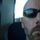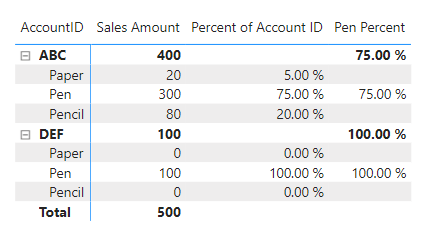cancel
Showing results for
Did you mean:

Fabric is Generally Available. Browse Fabric Presentations. Work towards your Fabric certification with the Cloud Skills Challenge.Regular Visitor

## Creating a custom Percentage column in Direct Query mode

I have a dataset imported from my DB2 table which looks like the following:

 AccountID Category Amount ABC Pen 100 ABC Paper 20 ABC Pencil 80 ABC Total 200 DEF Pen 100 DEF Paper 0 DEF Pencil 0 DEF Total 100

I need to display just the Total row for every Account in a table visual but need to create 3 columns- Pen % , Pencil %, Paper% which depict the percentage spent on that item by that particular account ID.

I was able to create a query using DAX when i used Excel to import this data:
Pen Percentage = IF('stationary'[Category]="Total", CALCULATE(SUM('stationary'[Amount]),FILTER('stationary', 'stationary'[Category]<>"Total" && 'stationary'[AccountID] = EARLIER('stationary[AccountID]) && 'stationary'[Category]="Pen")) / 'stationary'[Amount] * 100 & "%", BLANK())

This query worked for me but it doesn't when i use DirectQuery mode. How can i achieve the same result?

1 ACCEPTED SOLUTIONSuper User

I don't know you are going to be able to do this with Direct Query. It has limitations, but if you simplify your DAX it might help.

Try the following measure. This will return the total percents correctly:

``````Percent of Account ID =
VAR varCategory = SELECTEDVALUE('Sales'[Category])
VAR varAccountID = SELECTEDVALUE('Sales'[AccountID])
VAR varTotal =
CALCULATE(
[Sales Amount],
ALLEXCEPT('Sales', 'Sales'[AccountID])
)
VAR varCategorySales =
CALCULATE(
[Sales Amount],
'Sales'[AccountID] = varAccountID,
'Sales'[Category] = varCategory
)
VAR Result = DIVIDE(varCategorySales, varTotal, BLANK())
RETURN
Result``````

Then for just the Pen percent, use this:

``````Pen Percent =
CALCULATE(
[Percent of Account ID],
KEEPFILTERS( Sales[Category] = "Pen" )
)``````

I used a matrix, and I got rid of the totals in Power Query. Let the visual give you the totals.Get the basics working first and see if the engine can generate the SQL code necessary for Direct Query. Then you can add IF statements or ISINSCOPE statements to get the visual like you want.

However, as a rule, I strongly advise against Direct Query. It has it uses, but import is where DAX works best, and if this were a multi-million record dataset, I don't care what DAX you write that gives you the results you want, it will be 3-10 times slower than an import model.

Did my answers help arrive at a solution? Give it a kudos by clicking the Thumbs Up!

DAX is for Analysis. Power Query is for Data Modeling

Proud to be a Super User!

MCSA: BI ReportingSuper User

I don't know you are going to be able to do this with Direct Query. It has limitations, but if you simplify your DAX it might help.

Try the following measure. This will return the total percents correctly:

``````Percent of Account ID =
VAR varCategory = SELECTEDVALUE('Sales'[Category])
VAR varAccountID = SELECTEDVALUE('Sales'[AccountID])
VAR varTotal =
CALCULATE(
[Sales Amount],
ALLEXCEPT('Sales', 'Sales'[AccountID])
)
VAR varCategorySales =
CALCULATE(
[Sales Amount],
'Sales'[AccountID] = varAccountID,
'Sales'[Category] = varCategory
)
VAR Result = DIVIDE(varCategorySales, varTotal, BLANK())
RETURN
Result``````

Then for just the Pen percent, use this:

``````Pen Percent =
CALCULATE(
[Percent of Account ID],
KEEPFILTERS( Sales[Category] = "Pen" )
)``````

I used a matrix, and I got rid of the totals in Power Query. Let the visual give you the totals.Get the basics working first and see if the engine can generate the SQL code necessary for Direct Query. Then you can add IF statements or ISINSCOPE statements to get the visual like you want.

However, as a rule, I strongly advise against Direct Query. It has it uses, but import is where DAX works best, and if this were a multi-million record dataset, I don't care what DAX you write that gives you the results you want, it will be 3-10 times slower than an import model.

Did my answers help arrive at a solution? Give it a kudos by clicking the Thumbs Up!

DAX is for Analysis. Power Query is for Data Modeling

Proud to be a Super User!

MCSA: BI ReportingAnnouncements#### Power BI Monthly Update - November 2023

Check out the November 2023 Power BI update to learn about new features.#### The largest Power BI and Fabric virtual conference

130+ sessions, 130+ speakers, Product managers, MVPs, and experts. All about Power BI and Fabric. Attend online or watch the recordings.Top Solution Authors
Top Kudoed Authors
Users online (1,760)